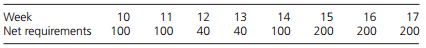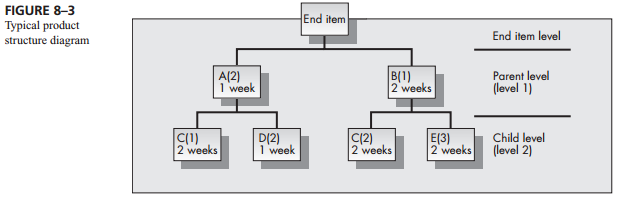### Create an Account

Home / Questions / Consider the product structure diagram given in Figure 8–3 Assume that the MPS for the e...

# Consider the product structure diagram given in Figure 8–3 Assume that the MPS for the end item for weeks 10 through 17 is Assume that lot-for-lot scheduling is used throughout Also assume that

Consider the product structure diagram given in Figure 8–3. Assume that the MPS for the end item for weeks 10 through 17 isAssume that lot-for-lot scheduling is used throughout. Also assume that there is no entering inventory in period 10 and no scheduled receipts.

a. Determine the planned order release for component A.

b. Determine the planned order release for component B.

c. Determine the planned order release for component C.Jul 28 2020 View more View LessSubscribe To Get Solution